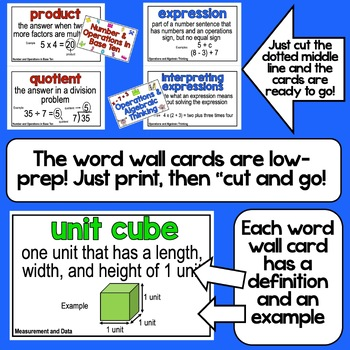# 5th Grade Math Word Wall5th, Homeschool
Subjects
Standards
Resource Type
Formats Included
• PDF
Pages
125 pages

### Description

Help your students master essential math vocabulary from the 5th Grade Common Core Math Standards. All the tools your students need are included! This pack includes 70+ vocabulary wall cards, 15 flip books with the vocabulary words grouped by topic, flashcards, and a printable personal math word wall for each student. This 125+ page printable math word wall pack includes everything you need to support 5th grade math vocabulary instruction. The included flip books are perfect for interactive notebooks or math folders!

This 5th grade math word wall pack contains the following:

• A printable 5th Grade Math Word Wall with 70+ words, color coded by domain PLUS printable domain labels for the math word wall in several styles
• 15 printable math vocabulary flip books for interactive math notebooks or reinforcement (words are grouped by domain and topic)
• Printable flashcards with all the math word wall words
• Math Toolkit labels (the flashcards can also be used to create a personal math glossary toolkit for each child called a Math Toolkit)

This resource was designed specifically for use with the Fifth Grade Common Core Standards. It is packed with items that will support 5th Grade math Standards vocabulary development.

If you are looking for a math word wall with a decorative border around each word card, check out the resources below:

5th Grade Common Core Math Word Wall and More (Chevron Edition)

5th Grade Common Core Math Word Wall and More (Polka Dot Edition)

5th Grade Common Core Math Word Wall and More (Sunburst Edition)

5th Grade Common Core Math Word Wall and More (Animal Print Edition)

5th Grade Common Core Math Word Wall and More (Camouflage Edition)

If you need a similar product to support the ELA Common Core Standards check out the resource below:

5th Grade Common Core ELA Ultimate Vocabulary Resource

If you are looking for math word walls for other grade levels check out the links below:

3rd Grade Common Core Math Vocabulary

4th Grade Common Core Math Vocabulary

6th Grade Common Core Math Vocabulary

Total Pages
125 pages
N/A
Teaching Duration
Lifelong tool
Report this Resource to TpT
Reported resources will be reviewed by our team. Report this resource to let us know if this resource violates TpT’s content guidelines.

### Standards

to see state-specific standards (only available in the US).
Use parentheses, brackets, or braces in numerical expressions, and evaluate expressions with these symbols.
Write simple expressions that record calculations with numbers, and interpret numerical expressions without evaluating them. For example, express the calculation “add 8 and 7, then multiply by 2” as 2 × (8 + 7). Recognize that 3 × (18932 + 921) is three times as large as 18932 + 921, without having to calculate the indicated sum or product.
Generate two numerical patterns using two given rules. Identify apparent relationships between corresponding terms. Form ordered pairs consisting of corresponding terms from the two patterns, and graph the ordered pairs on a coordinate plane. For example, given the rule “Add 3” and the starting number 0, and given the rule “Add 6” and the starting number 0, generate terms in the resulting sequences, and observe that the terms in one sequence are twice the corresponding terms in the other sequence. Explain informally why this is so.
Add and subtract fractions with unlike denominators (including mixed numbers) by replacing given fractions with equivalent fractions in such a way as to produce an equivalent sum or difference of fractions with like denominators. For example, 2/3 + 5/4 = 8/12 + 15/12 = 23/12. (In general, 𝘢/𝘣 + 𝘤/𝘥 = (𝘢𝘥 + 𝘣𝘤)/𝘣𝘥.)
Solve word problems involving addition and subtraction of fractions referring to the same whole, including cases of unlike denominators, e.g., by using visual fraction models or equations to represent the problem. Use benchmark fractions and number sense of fractions to estimate mentally and assess the reasonableness of answers. For example, recognize an incorrect result 2/5 + 1/2 = 3/7, by observing that 3/7 < 1/2.